Geometry 7-4 Similarity in Right Triangles
starstarstarstarstarstarstarstarstarstar
by Matthew Richardson
| 27 Questions
Note from the author:
A complete formative lesson with embedded slideshow, mini lecture screencasts, checks for understanding, practice items, mixed review, and reflection. I create these assignments to supplement each lesson of Pearson's Common Core Edition Algebra 1, Algebra 2, and Geometry courses. See also mathquest.net and twitter.com/mathquestEDU.
The outlined content above was added from outside of Formative.
The outlined content above was added from outside of Formative.1
2
1
10 pts
Solve It! Draw a diagonal of a rectangular piece of paper to form two right triangles. In one triangle, draw the altitude from the right angle to the hypotenuse. Number the angles as shown. Cut out the three triangles.

How can you match the angles of the triangles to show that all three triangles are similar?
2
10 pts
Solve It! Explain how you know the matching angles are congruent.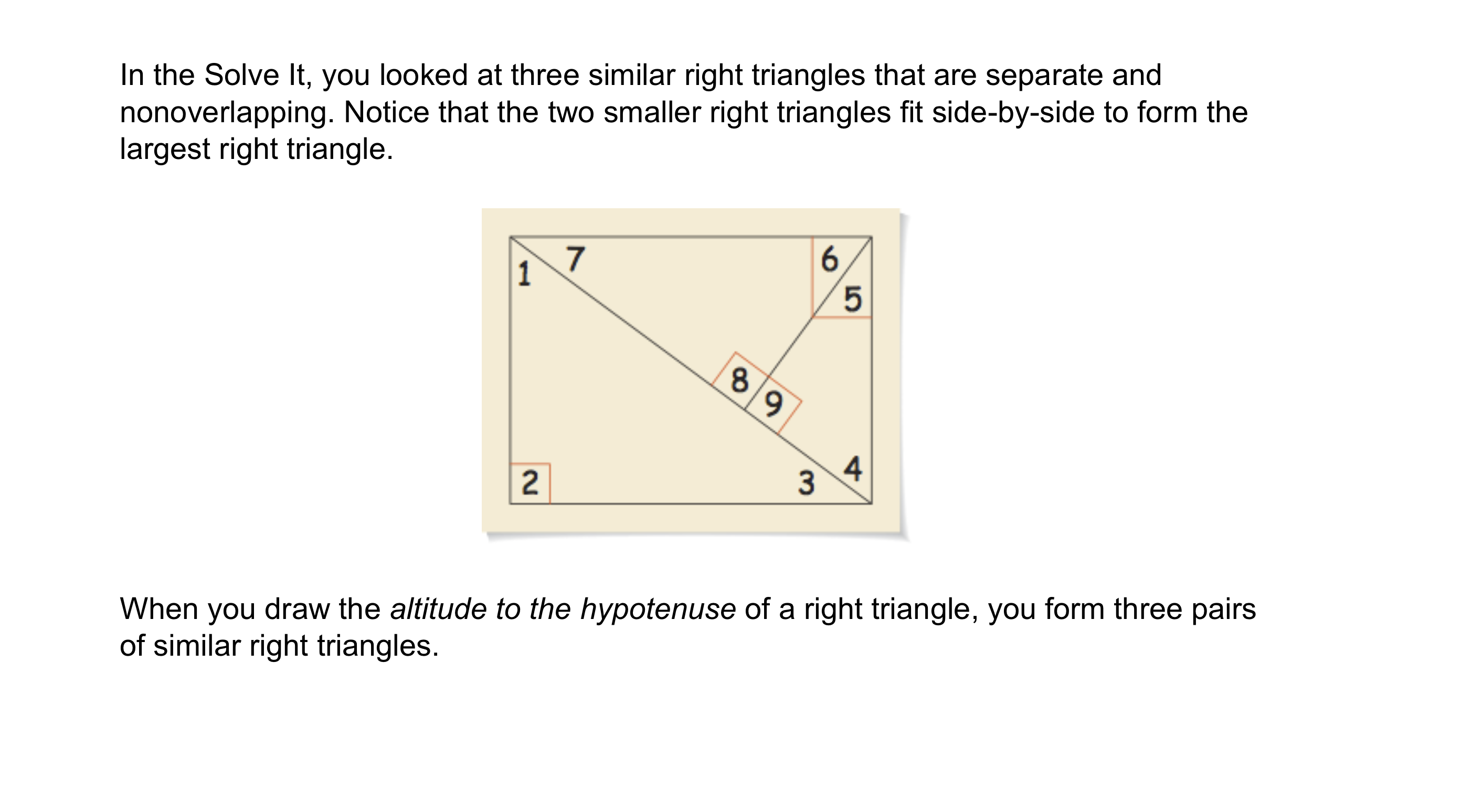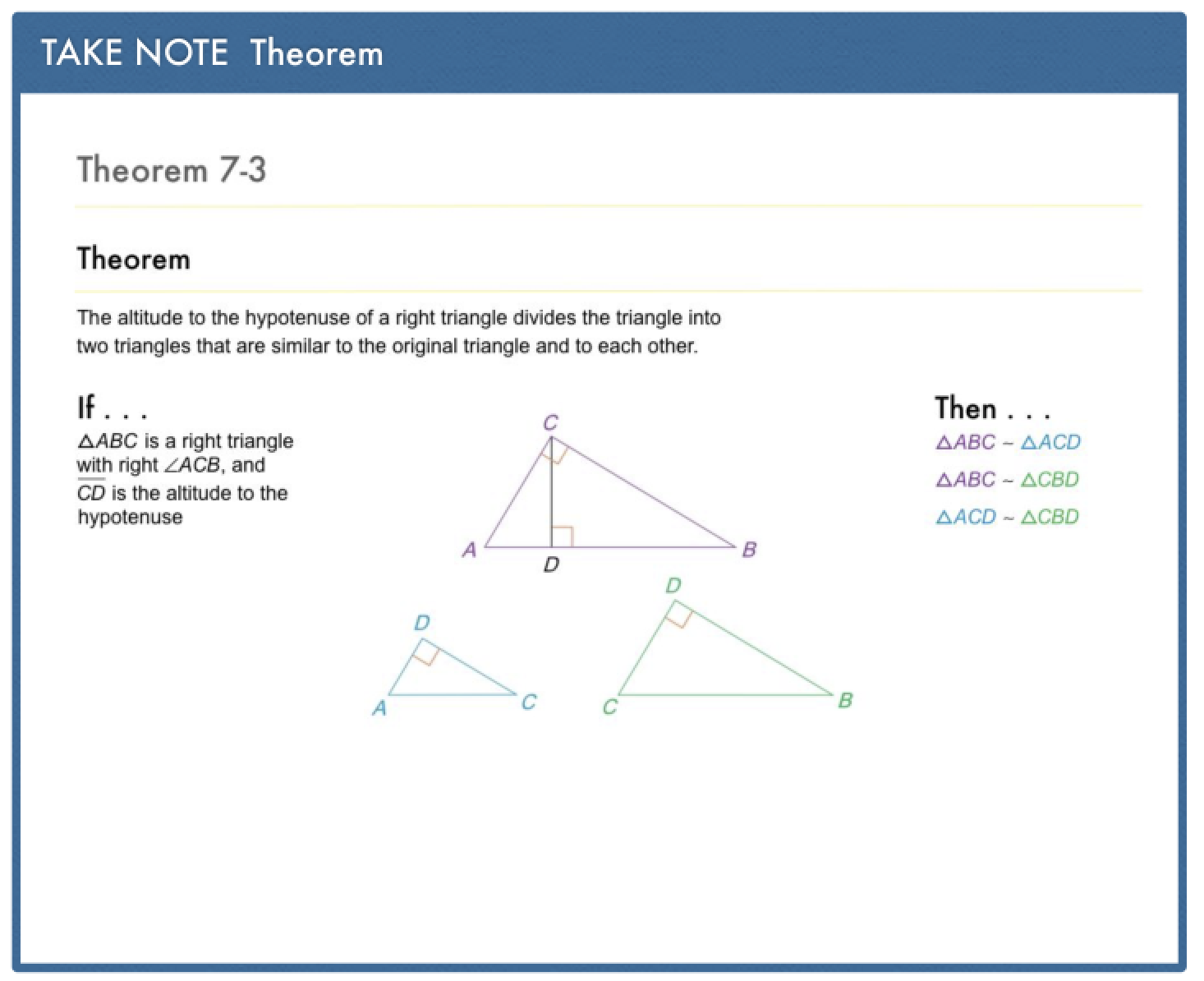The outlined content above was added from outside of Formative.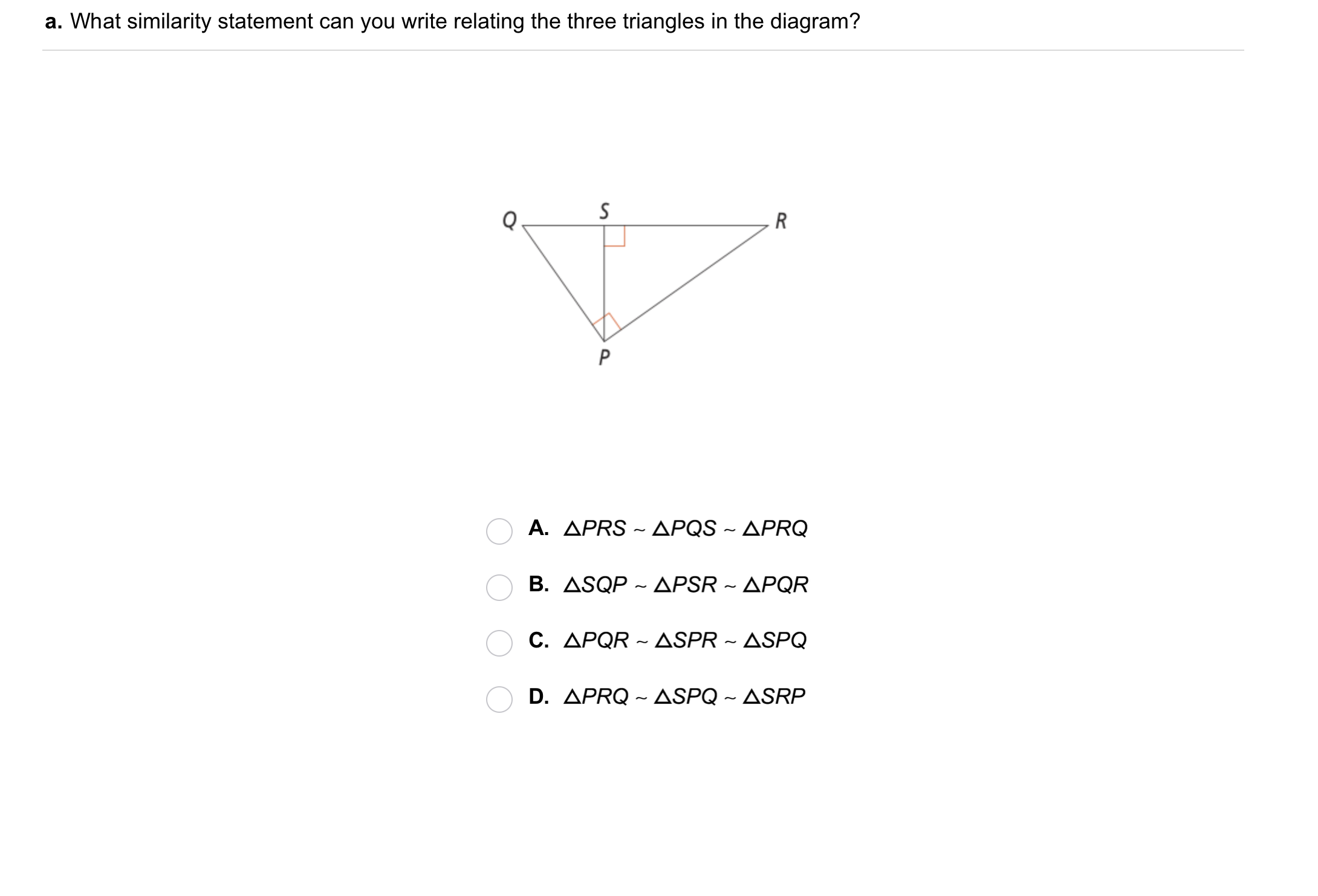3
3
10 pts
Problem 1 Got It?
A
B
C
D4
4
10 pts
Problem 1 Got It?
A
B
C
DThe outlined content above was added from outside of Formative.5
5
10 pts
Problem 2 Got It?
A
B
C
D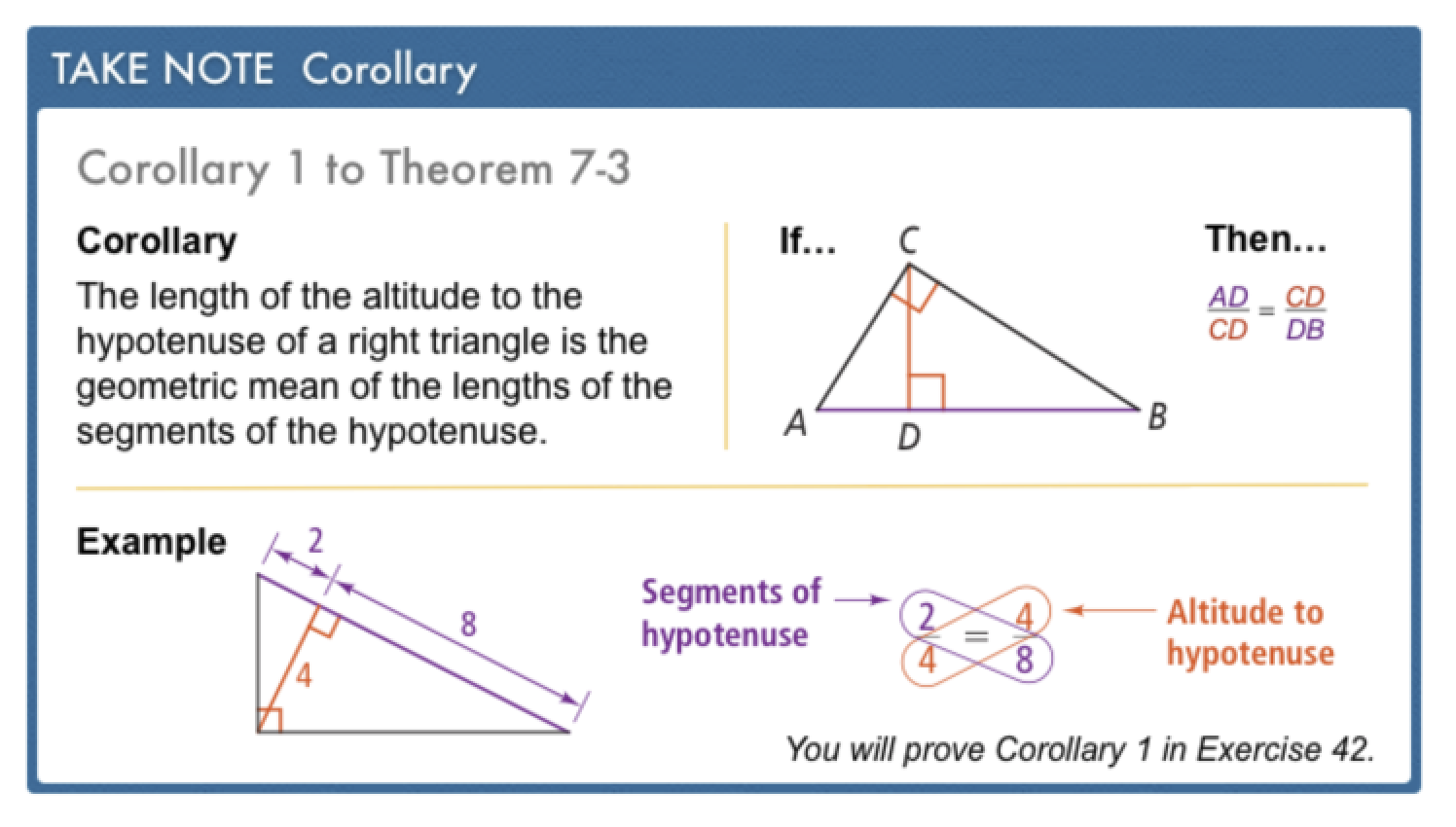The outlined content above was added from outside of Formative.6
6
10 pts
Problem 3 Got It?
A
B
C
D
The outlined content above was added from outside of Formative.7
7
10 pts
Problem 4 Got It? From point D, the robot must turn right and move to point B to put the bottle in the recycling bin. How many inches does the robot travel from D to B? Enter only the number.8
8
10 pts
What is the geometeric mean of 4 and 9?
A
B
C
D9
9
10 pts
What is the geometric mean of 4 and 12?
A
B
C
D10
10
10 pts
A
B
C
D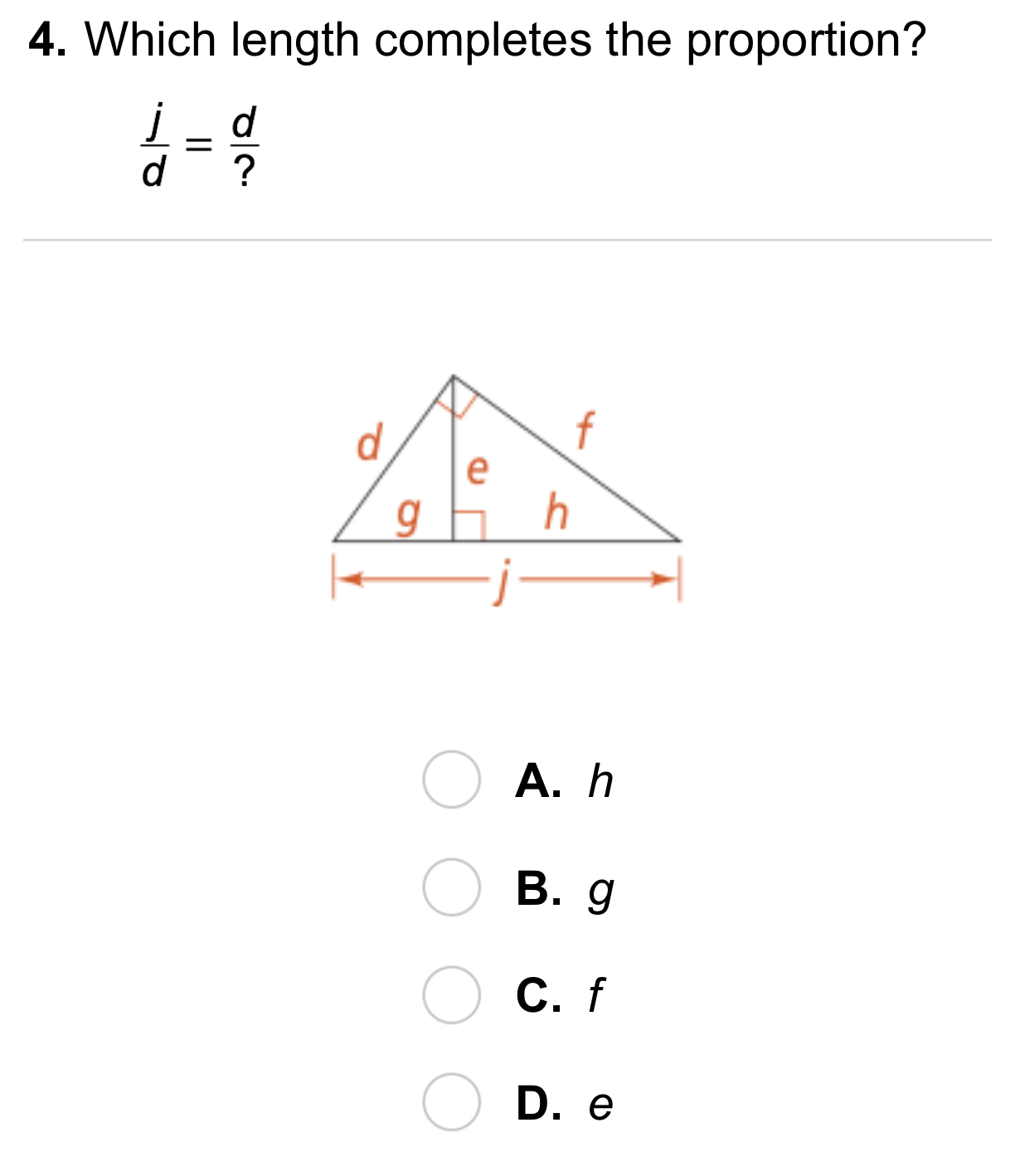11
11
10 pts
A
B
C
D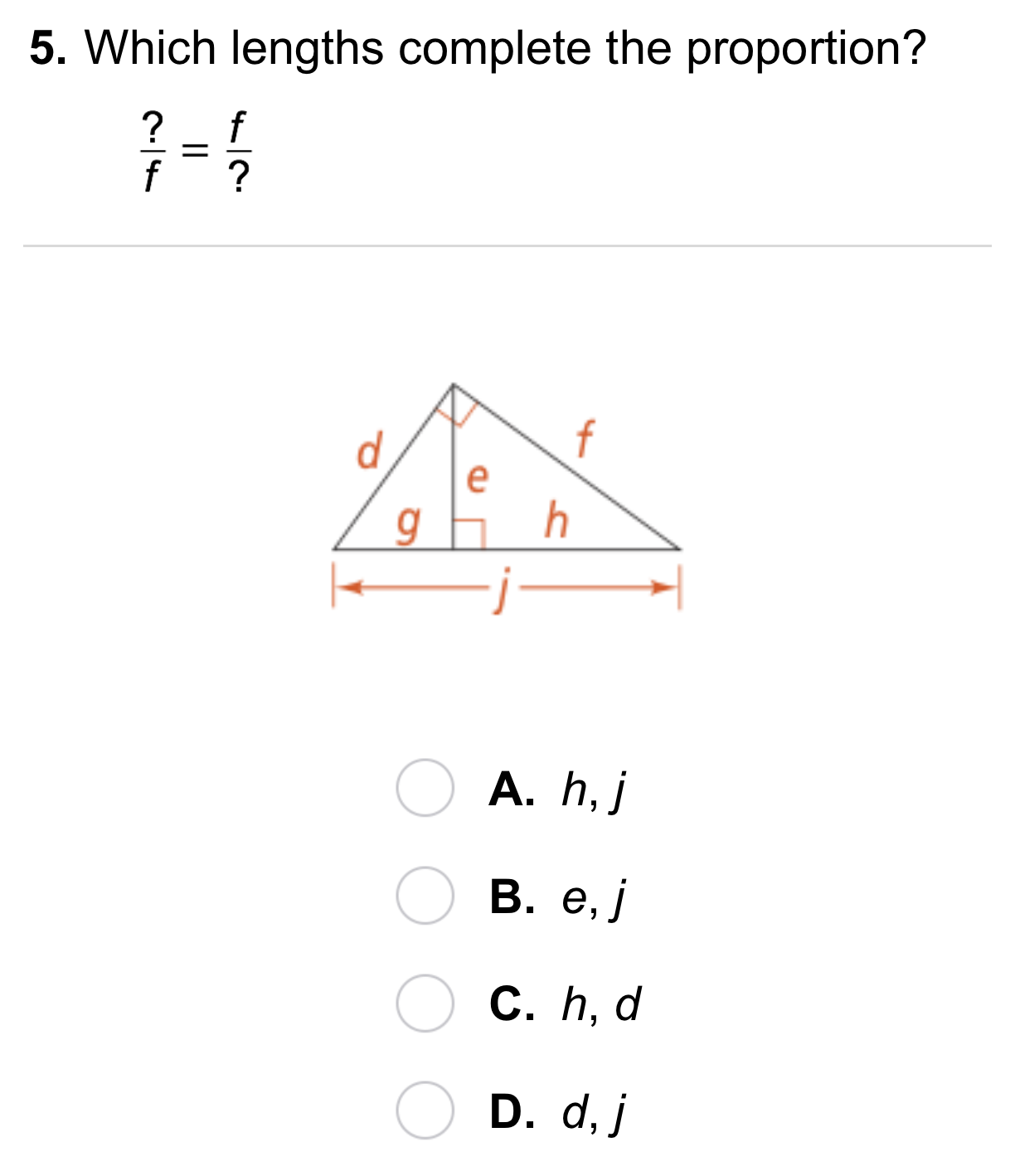12
12
10 pts
A
B
C
D13
13
10 pts
A
B
C
D14
14
10 pts
Vocabulary: Classify the segments of △RST. Sides may have more than one classification.
• segment RP
• segment RT
• segment TP
• The hypotenuse
• The segments of the hypotenuse
• The segment of the hypotenuse adjacent to leg ST15
15
10 pts
Error Analysis: A classmate wrote an incorrect proportion to find x. Explain and correct the error.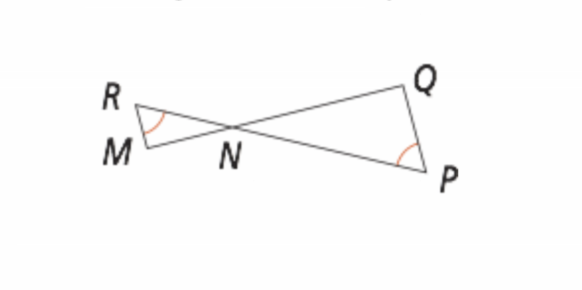16
17
16
10 pts
Review Lesson 7-3: Write a similarity statement for the two triangles.
△RMN ~ △PNQ
△RMN ~ △NQP
△NMR ~ △QPN
△NRM ~ △NPQ
17
10 pts
Review Lesson 7-3: How do you know the triangles are similar?18
19
18
10 pts
Review Lesson 6-2: Algebra: Find the value of x in ▱RSTV.
19
10 pts
Review Lesson 6-2: Algebra: Find the value of y in ▱RSTV.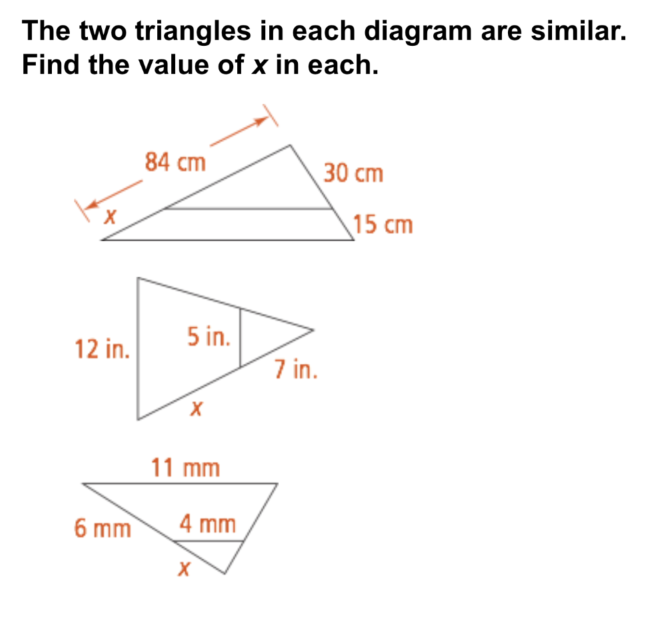20
21
22
20
10 pts
Review Lesson 7-2: Enter only the value of x.
21
10 pts
Review Lesson 7-2: Enter only the value of x.
22
10 pts
Review Lesson 7-2: Enter only the value of x. Round to the nearest hundredth.23
10 pts
Vocabulary Review: Complete each sentence with the correct word from the list.
• parallel
• perpendicular
• congruent
• isosceles
• The altitude of a triangle is a segment from a vertex to the opposite side that is __?__ to the opposite side.
• In an isosceles triangle, the altitude to the base divides the triangle into two __?__ triangles.24
24
10 pts
Vocabulary Review: Identify the altitude of △ABC.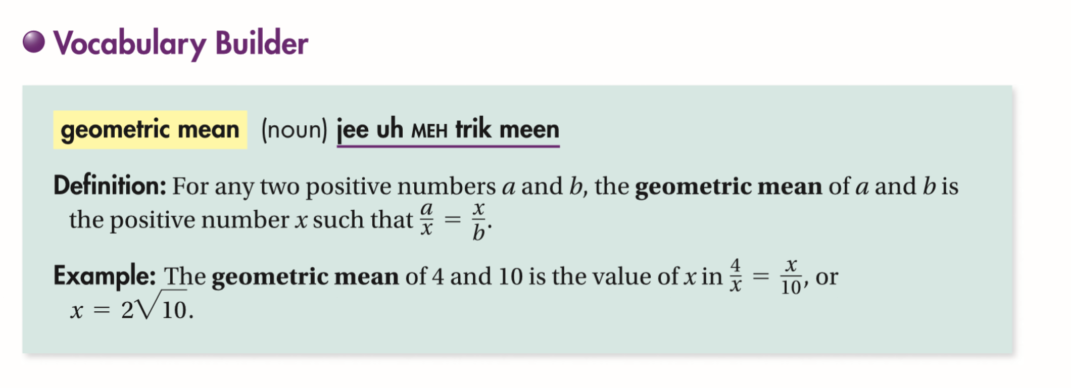25
10 pts
Use Your Vocabulary: Which proportion can you use to find the geometric mean of 5 and 15?

26
15 pts
Use Your Vocabulary: Complete the items on the canvas.
27
10 pts
Reflection: Math Success
Add to my formatives list

Formative uses cookies to allow us to better understand how the site is used. By continuing to use this site, you consent to the Terms of Service and Privacy Policy.